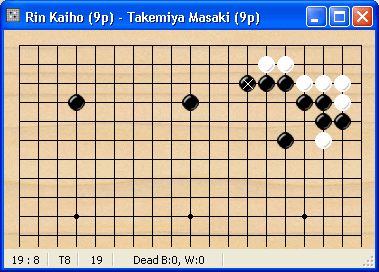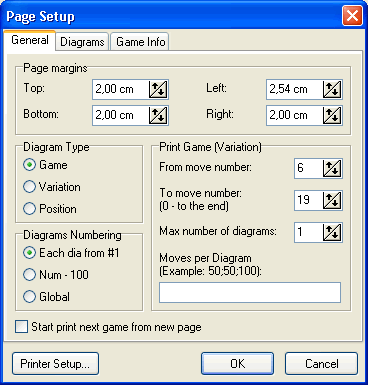# Print variation

Actually, this mode is subset of Print game mode.

So if you want print only one variation from fuseki/joseki or part of the game:

1. Set Page Setup->General->Diagram Type to Game.

2. Set Page Setup->General->From Move Number to the number from which you want have numbering in the Diagram (6 in example).

3. Set Page Setup->General->To Move Number to the number where variation finish (19 in example).

4. Set Page Setup->General->Max Number of diagrams to 1 if you want 1 diagram printout.

5. Set Page Setup->General->Diagrams Numbering to Each dia from #1 (in example) if you want start numbering from 1

6. Set diagram size and so on in Page Setup->Diagrams.

7. Switch of game header output in Page Setup->Game Info

Example. Let's print variation from half board fuseki:Suppose, we want to print variation from the white kakari (move #6) to the move 19 (to the position shown here). We want start numbering from 1 and print all in one diagram.

So we have to set Page Setup->General options like shown below.Print preview of the diagram:Numbers on header 1 - 14 (6 -19) means: 1 - 14: 1st and last moves in the diagram; (6 - 19) - absolute values (i.e. from the begin of game) for the correspondence numbers.